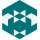Home Communities
IT Knowledge
Inspiration
Languages
EN

# JavaScript - check words similarity (fuzzy compare with bigrams)

11 points
Created by:Dexter
510

In this article, we would like to show how to check words similarity in JavaScript.

Below logic:

1. calculates words bigrams,
2. counts bigram hits to find similarity,
3. divides hits by bigrams to calculate final words similarity.

Below `checkSimilarity()` function result indicates how two words are similar.

Similarity measured is from `0` where:

• `0` - means: the worlds are totally different,
• `>=1` - means: the words are the same or contain similar part.

That kind of approach is may be applied in fuzzy search.

Practical example:

``````// ONLINE-RUNNER:browser;

const createBigram = word => {
const input = word.toLowerCase();
const vector = [];
for (let i = 0; i < input.length; ++i) {
vector.push(input.slice(i, i + 2));
}
return vector;
};

const checkSimilarity = (a, b) => {
if (a.length > 0 && b.length > 0) {
const aBigram = createBigram(a);
const bBigram = createBigram(b);
let hits = 0;
for (let x = 0; x < aBigram.length; ++x) {
for (let y = 0; y < bBigram.length; ++y) {
if (aBigram[x] === bBigram[y]) {
hits += 1;
}
}
}
if (hits > 0) {
const union = aBigram.length + bBigram.length;
return (2.0 * hits) / union;
}
}
return 0;
};

// Usage example:

console.log(checkSimilarity('Chris',  'Chris'));  // 1
console.log(checkSimilarity('John1',  'John2'));  // 0.6
console.log(checkSimilarity('Ann',    'Matt' ));  // 0``````

Note: do not compare sentences or whole texts using the above function - it may lead to comparison mistakes.

## Alternative titles

Join to our subscribers to be up to date with content, news and offers.